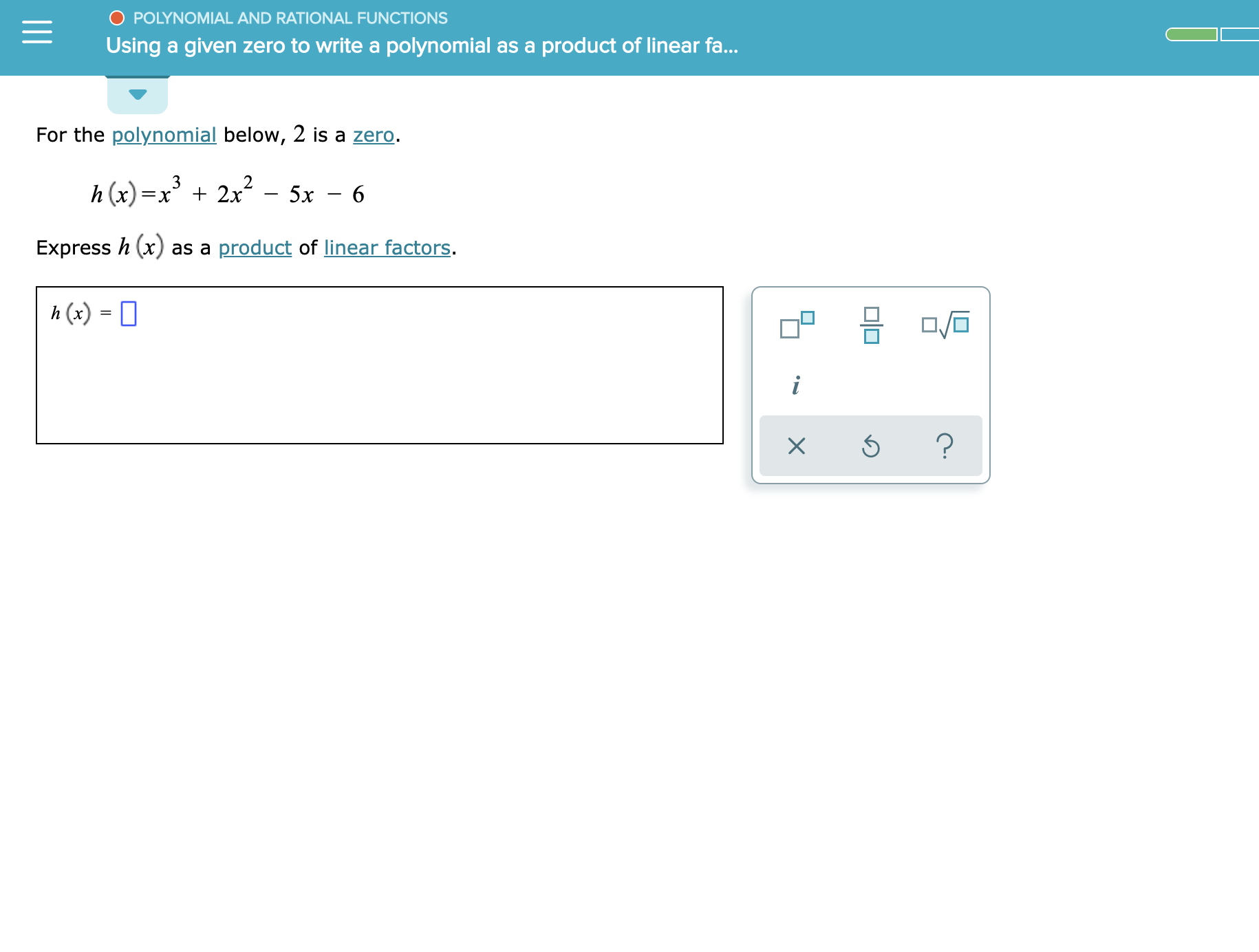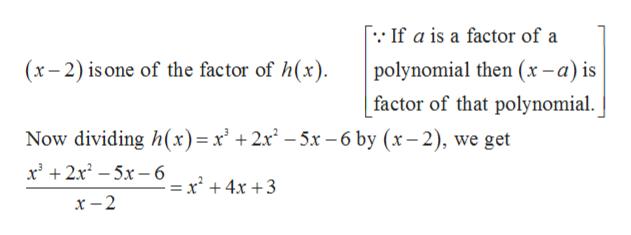O POLYNOMIAL AND RATIONAL FUNCTIONSUsinggiven zero to write a polynomial as aproduct of linear fa...аFor the polynomial below, 2 is a zero.2x-5xh(x)=XExpress h (x) as a product of linear factors.h (x) =i?

Question

See attachmenthelp_outlineImage TranscriptioncloseO POLYNOMIAL AND RATIONAL FUNCTIONS Using given zero to write a polynomial as a product of linear fa... а For the polynomial below, 2 is a zero. 2x-5x h(x) =X Express h (x) as a product of linear factors. h (x) = i ? fullscreen
Step 1

Given,

Step 2

Therefore,help_outlineImage TranscriptioncloseIf a is a factor of a (x-2) is one of the factor of h(x) polynomial then (x-a) is factor of that polynomial Now dividing h(x)=x' +2x2 -5x -6 by (r-2), we get 2x25x6- 2 + 4x + 3 x -2 fullscreen
Step 3

Now factorizing the obtained qu...

Want to see the full answer?

See Solution

Want to see this answer and more?

Our solutions are written by experts, many with advanced degrees, and available 24/7

See Solution
Tagged in

Other